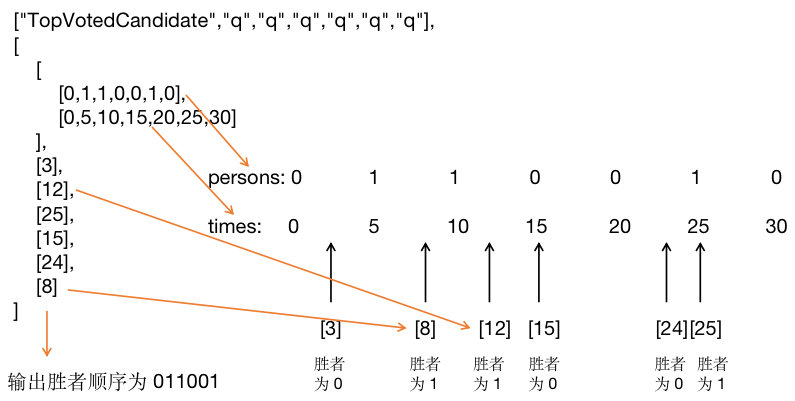LeetCode 911. 在线选举题目样例图解Java 解法，通过 96/97，真的吐槽时间。

class TopVotedCandidate {

private int[] persons;
private int[] times;
// 将每一次投票的获胜者保存下来，key为时间，value为候选人
private HashMap<Integer, Integer> winner = new HashMap<>();

public TopVotedCandidate(int[] persons, int[] times) {
this.persons = persons;  // 选票选择的候选人
this.times = times;  // 选票投出的时间

int[] votes = new int[persons.length + 1]; // 记录每个候选人的得票
int currentWinner = persons;  // 记录第i次投票获胜的候选人

for(int i = 0; i < times.length; i++){
// 票投给了其他人，考察是否需要改变获胜者
if(persons[i] != currentWinner) {
currentWinner = persons[i];
}
winner.put(times[i], currentWinner);
}
}

public int q(int t) {
if(winner.size() ==1) return persons;
// 二分查找 t 位于哪个时间区间
int l = 0, r = times.length - 1, index = -1;
while(l < r){
int mid = (r + l)/2;
if(times[mid] == t) {index = mid;break;}
else if(times[mid] > t) r = mid - 1;
else l = mid + 1;
}
if(index == -1) index =  times[r] > t ? r - 1 : r;
return winner.get(times[index]);
}
}